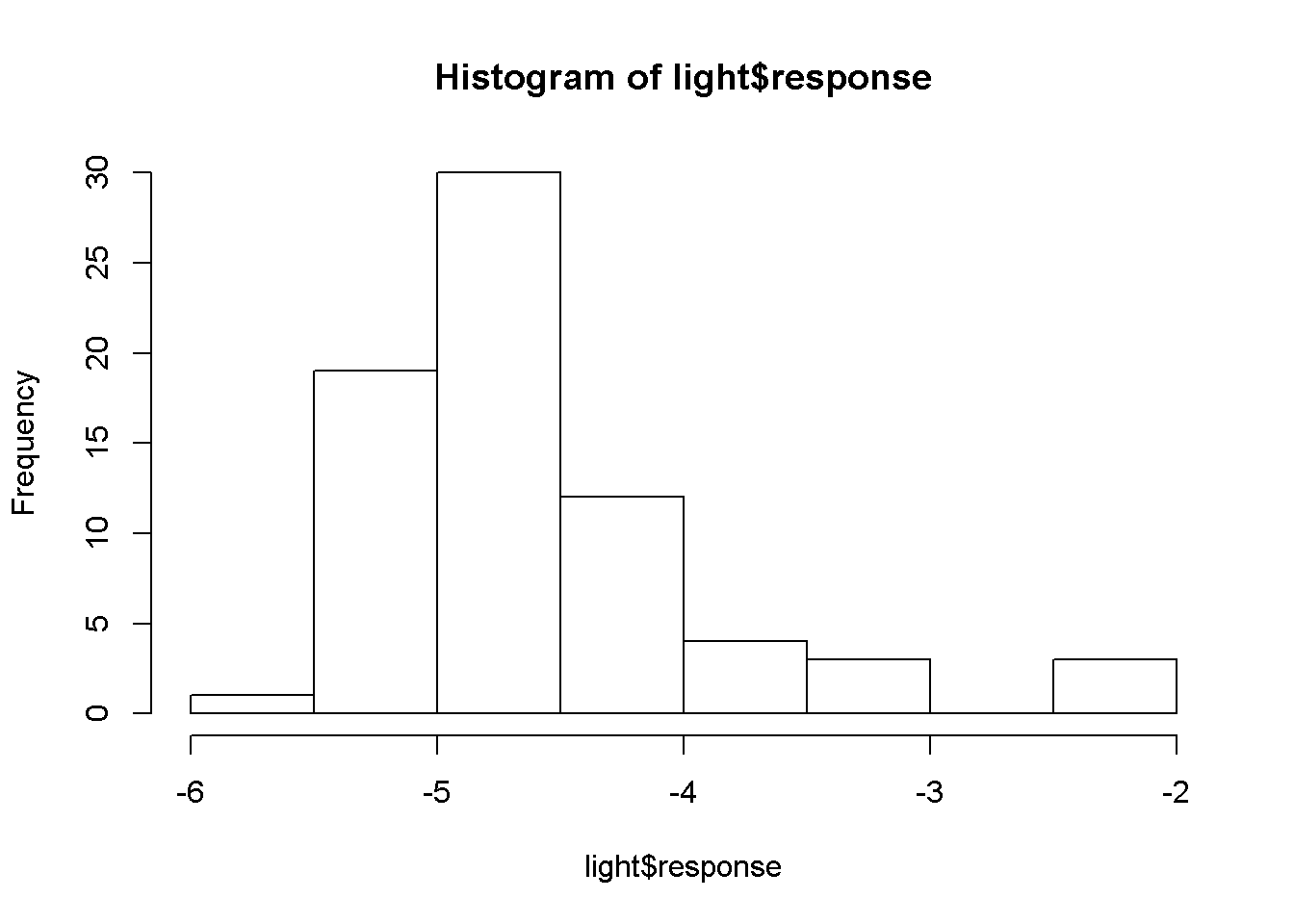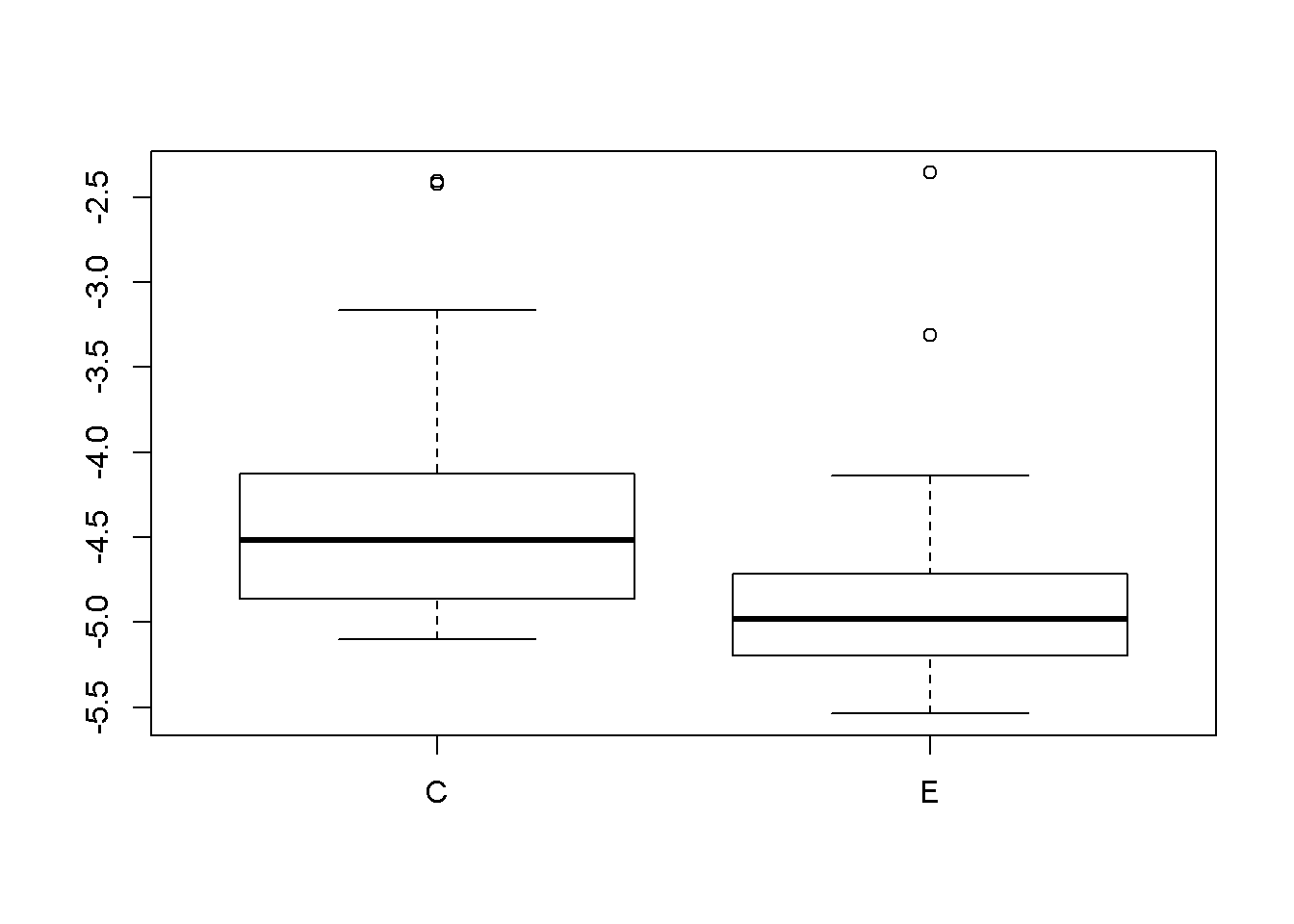Functions used in this Lab

• list.files()
• ls()
• boxplot
• subset
• hist
• par(mfrow = c(1,2))
• tapply

Outline of Lab

• load data from an .RData file
• examine dataframe
• plot boxplot
• split data into groups w/subset
• plot histograms
• calculate means and sd w/ tapply
• plot means w/error bars

1) Set working directory

I use the RStudio menu to select my working directory.

• Session
• Set working Directory
• Choose Directory
• Choose appropriate directory
setwd("C:/Users/lisanjie2/Desktop/TEACHING/1_STATS_CalU/1_STAT_CalU_2016_by_NLB/Lab/Lab6_review")

2) Check that the data I want is in the directory

I am going to work with a file called light.RData. I can use list.files() to check that the working directory that I have set contains that file.

list.files()
##   "finchs.csv"
##   "FUNCTIONplot2means.R"
##   "Lab_6_basic_data_analysis_review.html"
##   "Lab_6_basic_data_analysis_review.Rmd"
##   "Lab_6_basic_data_analysis_review_INCLASS_EXERCISE.html"
##   "Lab_6_basic_data_analysis_review_INCLASS_EXERCISE.Rmd"
##   "light.RData"
##   "plot2means.jpg"
##   "plot2means.png"
##  "plot2means.RData"
##  "rsconnect"
##  "subset.jpg"
##  "tapply.jpg"

3) Load Data stored as an .RData file

R can save data into its own species format. Here, I have saved data from a spreadsheet into an .RData file. This data can be loaded into my R session using the load() command. NOTE: the name of the file has to be in parentheses.

4) Check that the data is loaded into the R session

I can confirm that the data is loaded using the ls() command. The file “light.Rdata” loads an R object called “light”

ls()
##  "light"

5) Examine the data

I can use the standard command head, tail, and summary to check out the data.

##     treatment  response
## 869         C -4.504766
## 871         C -3.164909
## 873         C -4.320002
## 875         C -4.486886
## 877         C -4.207244
## 879         C -4.591058
tail(light)
##      treatment  response
## 1002         E -3.310792
## 1004         E -4.135877
## 1006         E -5.112481
## 1008         E -4.780870
## 1010         E -4.822527
## 1012         E -4.761931
summary(light)
##  treatment    response
##  C:36      Min.   :-5.537
##  E:36      1st Qu.:-5.026
##            Median :-4.758
##            Mean   :-4.592
##            3rd Qu.:-4.330
##            Max.   :-2.357

6) The light data

These are data from deer exclusion study at Trillium Trail in Pittsburgh, PA. C = control plots, E = exclusion plots. The numeric response variable is the ratio of sunlight at the forest floor to sunlight above the canopy. Actually, its the log of that ratio, eg log(light below / light above). The hypothesis is that when deer are excluded, vegetation increases in density and therefore light reachign the forest floor decreases.

7) Basic examination of raw data with a histogram

The data is organized into groups so we will want to split it up for our real analysis. HOwever, it can be informative just to look at the raw data with a histogram.

hist(light\$response)The data are skewed. Such is life.

8) Boxplots

The boxplot() function naturally handles grouped data so was can easily visualize these dat.

boxplot(response ~ treatment, data = light)Light levels are higher in the “C” treatment (the control where deer have access and can eat the vegetation)

9) Split the data with the subset() command

These data are split into two groups. Some rows of data are from control plots (“C”) and some are from exclosures (“E”). The boxplot() function naturally handles data structured like this. (The t.test function also does, but we won’t work with that today).

Not not all R functions handle grouped data so easily. We’ll use the subset() command to split the data into two seperate R objects that we can then work with seperately.

The subset command

The subset() command takes three arguements

• the data to be subset (out “light” data object)
• a command for how to subset the data
• the columns to return in the final output

The command we will use to subset the data is “treatment ==”C" “, which tells the subset command,”look at the treatment column and give me just those rows that have “C” in them“.

We use the arguement “select = c(”treatment“,”response“)” to tell subset to give us both the treatment and response columns in our output.

Annotated subset() command

The picture below is an annotated version of the subset command, though applied to a differen dataset, not the light data.